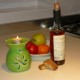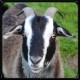# 2255. Goldilocks breaks in again

Goldilocks saw the Three Bears shopping in town so she headed for their house.  There were three plates of grits on the table. She tried each dish. The first dish was too hot, the second was too cold, but the third was just right so she gobbled it up.

Next she sat in a chair. Clearly she had eaten the grits while standing up. The first chair was too big, the second was too small, but the third was just right but it soon collapsed because she had eaten too much.

Next she went into the bedroom to have a rest. The first bed was too lumpy, the second was too bumpy, and the third was too grumpy. Oh goodness me! Oh hell’s bells! Oh shock and horror! She had gone to the wrong house. The Three Giraffes were still in bed.

## 19 thoughts on “2255. Goldilocks breaks in again”

1.Yvonne

Oh well, what’s a little breach of copyright between friends?

Liked by 1 person

2.Herb

That’s what you get for listening to your GPS too closely.

Liked by 1 person

1.Bruce Goodman Post author

I hired a car – quite the latest – and the GPS in it didn’t even have my road listed (my road=where I live).

Liked by 1 person

3.dumbestblogger

When one takes into consideration the thermal mass of the bowl, the thermal mass of the porridge, the air temperature of the house and the heat of the porridge when it was removed from the stove how long ago would the porridge need to have been dished up in order for the temperature to be “just right.” Please show your work.

Liked by 1 person

1.Bruce Goodman Post author

((k+2) (1 – [wz + h + j – q]^2 – [(gk + 2g + k + 1)(h + j) + h – z]^2 – [16(k + 1)^3(k + 2)(n + 1)^2 + 1 – f^2]^2 -[2n + p + q + z – e]^2 -[e^3(e + 2)(a + 1)^2 + 1 – o^2]^2 -[(a^2 – 1)y^2 + 1 – x^2]^2 -[16r^2y^4(a^2 – 1) + 1 – u^2]^2 -[n + l + v – y]^2 -[(a^2 – 1)l^2 + 1 – m^2]^2 -[ai + k + 1 – l – i]^2 -[((a + u^2(u^2 – a))^2 – 1)(n + 4dy)^2 + 1 – (x + cu)^2]^2 -[p + l(a – n – 1) + b(2an + 2a – n^2 – 2n – 2) – m]^2 -[q + y(a – p – 1) + s(2ap + 2a – p^2 – 2p – 2) – x]^2 -[z + pl(a – p) + t(2ap – p^2 – 1) – pm]^2) – 1) – | (k+2) (1 – [wz + h + j – q]^2 – [(gk + 2g + k + 1)(h + j) + h – z]^2 – [16(k + 1)^3(k + 2)(n + 1)^2 + 1 – f^2]^2 -[2n + p + q + z – e]^2 -[e^3(e + 2)(a + 1)^2 + 1 – o^2]^2 -[(a^2 – 1)y^2 + 1 – x^2]^2 -[16r^2y^4(a^2 – 1) + 1 – u^2]^2 -[n + l + v – y]^2 -[(a^2 – 1)l^2 + 1 – m^2]^2 -[ai + k + 1 – l – i]^2 -[((a + u^2(u^2 – a))^2 – 1)(n + 4dy)^2 + 1 – (x + cu)^2]^2 -[p + l(a – n – 1) + b(2an + 2a – n^2 – 2n – 2) – m]^2 -[q + y(a – p – 1) + s(2ap + 2a – p^2 – 2p – 2) – x]^2 -[z + pl(a – p) + t(2ap – p^2 – 1) – pm]^2) – 1| = 0
Hope you’re happy now.

Liked by 1 person

1.dumbestblogger

Ah, I see that we have made a rather fundamental mistake. You have transcribed your units of measure as pints instead of seconds.

Liked by 1 person

4.Sarah Angleton

Well, at least giraffe’s are a better choice than bears. Giraffe’s will usually stick their necks out for you.

Liked by 1 person

1.Bruce Goodman Post author

I agree. And anteaters are no good because they stick their noses into everything.

Liked by 1 person